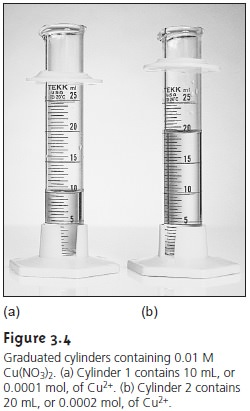Home | | Modern Analytical Chemistry | Classifying Analytical Techniques

# Classifying Analytical TechniquesAnalyzing a sample generates a chemical or physical signal whose magnitude is pro- portional to the amount of analyte in the sample.

Classifying Analytical Techniques

Analyzing a sample generates a chemical or physical signal whose magnitude is pro- portional to the amount of analyte in the sample. The signal may be anything we can measure; common examples are mass, volume, and absorbance. For our pur- poses it is convenient to divide analytical techniques into two general classes based on whether this signal is proportional to an absolute amount of analyte or a relative amount of analyte.Consider two graduated cylinders, each containing 0.01 M Cu(NO3)2 (Figure 3.4). Cylinder 1 contains 10 mL, or 0.0001 mol, of Cu2+; cylinder 2 contains 20 mL, or 0.0002 mol, of Cu2+. If a technique responds to the absolute amount of analyte in the sample, then the signal due to the analyte, SA, can be expressed as

SA = knA                                                                                     3.1

where nA is the moles or grams of analyte in the sample, and k is a proportionality constant. Since cylinder 2 contains twice as many moles of Cu2+ as cylinder 1, an- alyzing the contents of cylinder 2 gives a signal that is twice that of cylinder 1.

A second class of analytical techniques are those that respond to the relative amount of analyte; thus

SA = kCA           ..........3.2

where CA is the concentration of analyte in the sample. Since the solutions in both cylinders have the same concentration of Cu2+, their analysis yields identical signals.

Techniques responding to the absolute amount of analyte are called total analysis techniques. Historically, most early analytical methods used total analysis techniques, hence they are often referred to as “classical” techniques. Mass, volume, and charge are the most common signals for total analysis techniques, and the cor- responding techniques are gravimetry , titrimetry , and coulometry . With a few exceptions, the signal in a total analysis tech- nique results from one or more chemical reactions involving the analyte. These re- actions may involve any combination of precipitation, acid–base, complexation, or redox chemistry. The stoichiometry of each reaction, however, must be known tosolve equation 3.1 for the moles of analyte.

Techniques, such as spectroscopy , potentiometry , and voltammetry , in which the signal is proportional to the relative amount of analyte in a sample are called concentration techniques. Since most concentration techniques rely on measuring an optical or electrical signal, they also are known as “instrumental” techniques. For a concentration technique, the rela- tionship between the signal and the analyte is a theoretical function that depends on experimental conditions and the instrumentation used to measure the signal. For this reason the value of k in equation 3.2 must be determined experimentally.

Study Material, Lecturing Notes, Assignment, Reference, Wiki description explanation, brief detail
Modern Analytical Chemistry: The Language of Analytical Chemistry : Classifying Analytical Techniques |